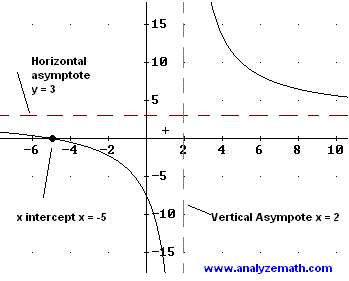# Write Rational Functions - Problems With Solutions

Find rational functions given their characteristics such as vertical asymptotes, horizontal asymptote, x intercepts, hole.

 Problem 1: Write a rational function f that has a vertical asymptote at x = 2, a horizontal asymptote y = 3 and a zero at x = - 5. Solution to Problem 1: Since f has a vertical is at x = 2, then the denominator of the rational function contains the term (x - 2). Function f has the form. f(x) = g(x) / (x - 2) g(x) which is in the numerator must be of the same degree as the denominator since f has a horizontal asymptote. Also g(x) must contain the term (x + 5) since f has a zero at x = - 5. Hence f(x) = 3 (x + 5) / (x - 2) Check that all the characteristics listed in the problem above are in the graph of f shown below.. Problem 2: Write a rational function g with vertical asymptotes at x = 3 and x = -3, a horizontal asymptote at y = -4 and with no x intercept. Solution to Problem 2: Since g has a vertical is at x = 3 and x = -3, then the denominator of the rational function contains the product of (x - 3) and (x + 3). Function g has the form. g(x) = h(x) / [ (x - 3)(x + 3) ] For the horizontal asymptote to exist, the numerator h(x) of g(x) has to be of the same degree as the denominator with a leading coefficient equal to -4. At the same time h(x) has no real zeros. Hence f(x) = [ -4x 2 - 6 ] / [ (x - 3)(x + 3) ] Check the characteristics in the graph of g shown below.. Problem 3: Write a rational function h with a hole at x = 5, a vertical asymptotes at x = -1, a horizontal asymptote at y = 2 and an x intercept at x = 2. Solution to Problem 3: Since h has a hole at x = 5, both the numerator and denominator have a zero at x = 5. Also the vertical asymptote at x = -1 means the denominator has a zero at x = -1. An x intercept at x = 2 means the numerator has a zero at x = 2. Finally the horizontal asymptote y = 2 means that the numerator and the denominator have equal degrees and the ratio of their leading coefficients is equal to 2. Hence h(x) = [ 2 (x - 5)(x - 2) ] / [ (x - 5)(x + 1) ] The graph of h is shown below, check the characteristics.. Problem 4: Write a rational function f with a slant asymptote y = x + 4, a vertical asymptote at x = 5 and one of the zeros at x = 2. Solution to Problem 4: The graph of f has a slant asymptote y = x + 4 and a vertical asymptote at x = 5, hence f(x) may be written as follows f(x) = (x + 4) + a / (x - 5) where a is a constant to be determined using the fact that f(2) = 0 since f has a zero at x = 2. f(2) = (2 + 4) + a / (2 - 5) = 0 Solve the above for a to obtain. a = 18 Hence f(x) is given by. f(x) = (x + 4) + 18 / (x - 5) = (x 2 - x - 2) / (x - 5) Check the characteristics of the graph of f shown below.. More math problems with detailed solutions in this site.